# The times that college students spend studying per week have a distribution skewed to the left...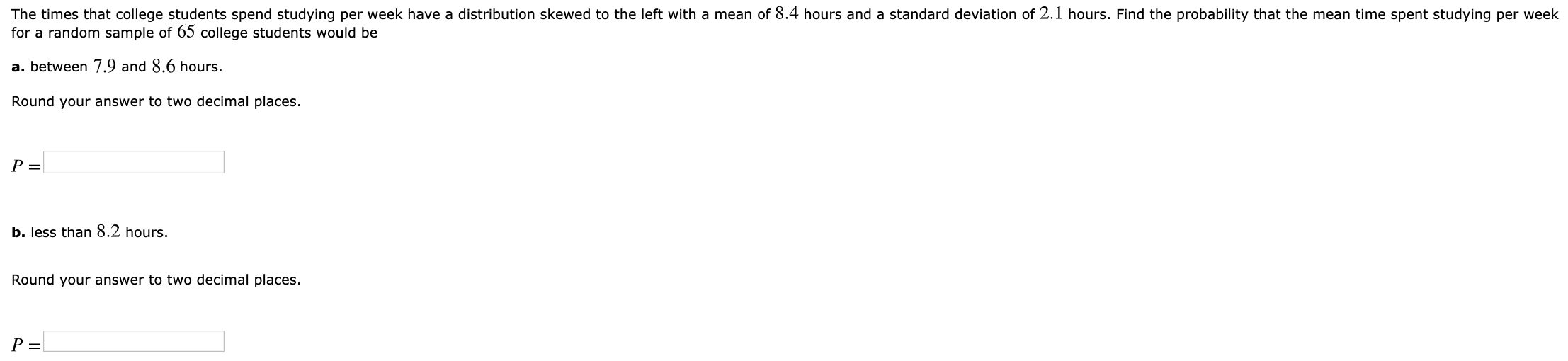The times that college students spend studying per week have a distribution skewed to the left with a mean of 8.4 hours and a standard deviation of 2.1 hours. Find the probability that the mean time spent studying per week for a random sample of 65 college students would be a. between 7.9 and 8.6 hours. Round your answer to two decimal places. P= b. less than 8.2 hours. Round your answer to two decimal places. P=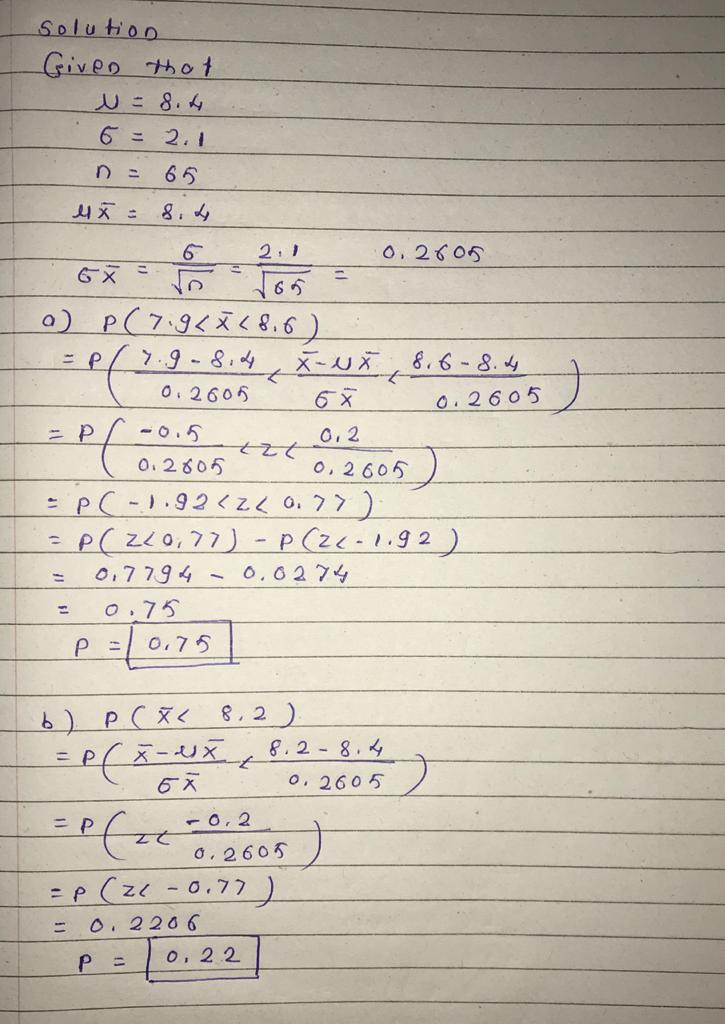#### Earn Coin

Coins can be redeemed for fabulous gifts.

Similar Homework Help Questions
• ### The times that college students spend studying per week have a distribution skewed to the right...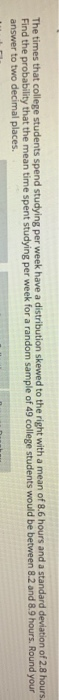The times that college students spend studying per week have a distribution skewed to the right with a mean of 8.6 hours and a standard deviation of 2.8 hours. Find the probability that the mean time spent studying per week for a random sample of 49 college students would be between 8.2 and 8.9 hours. Round your answer to two decimal places.

• ### Chapter 07, Section 7.4, Problem 036 The times that college students spend studying per week have...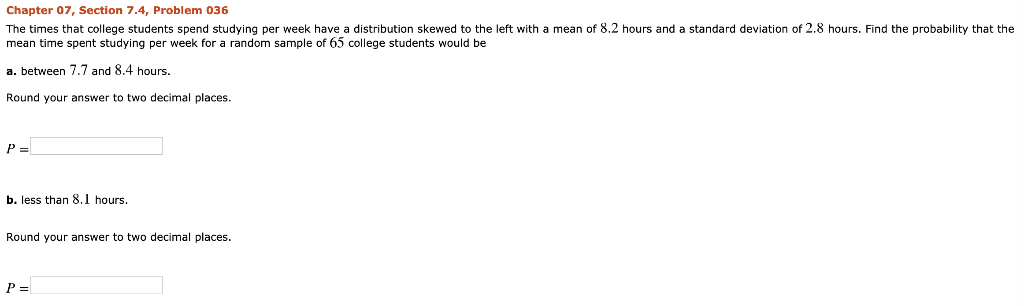Chapter 07, Section 7.4, Problem 036 The times that college students spend studying per week have a distribution skewed to the left with a mean of 8.2 hours and a standard deviation of 2.8 hours. Find the probability that the mean time spent studying per week for a random sample of 65 college students would be a. between 7.7 and 8.4 hours. Round your answer to two decimal places. b. less than 8.1 hours. Round your answer to two decimal...

• ### The times that college students spend studying per week have a distribution skewed to the right...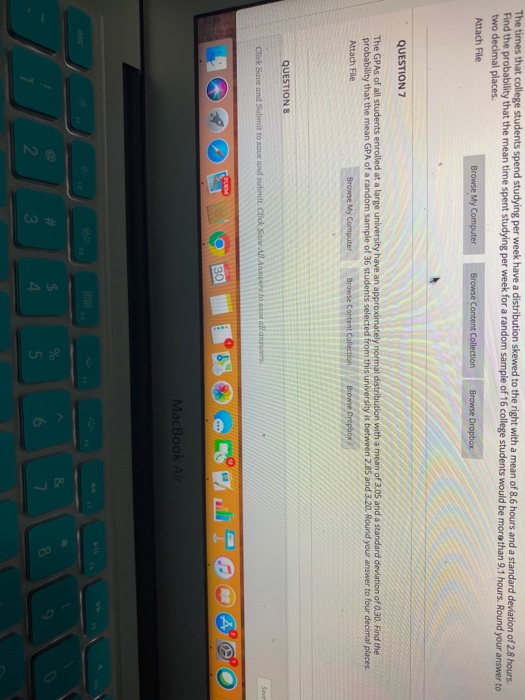The times that college students spend studying per week have a distribution skewed to the right with a mean of 8.6 hours and a standard deviation of 2.8 hours. Find the probability that the mean time spent studying per week for a random sample of 16 college students would be more than 9.1 hours. Round your answer to two decimal places. Attach File Browse My Computer Browse Content Collection Browse Dropbox QUESTION 7 The GPAs of all students enrolled at...

• ### LUULIS spend scuoying per week have a distribution skewed to the right with a mean of...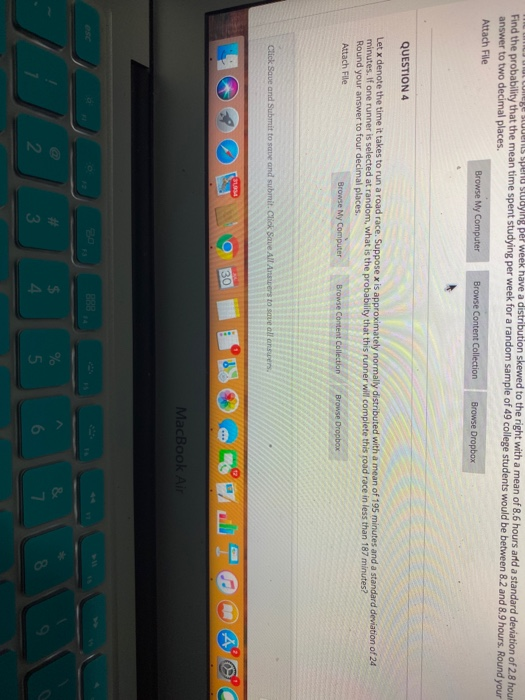LUULIS spend scuoying per week have a distribution skewed to the right with a mean of 8.6 hours and a standard deviation of 28 houm Find the probability that the mean time spent studying per week for a random sample of 49 college students would be between 8.2 and 8.9 hours. Round your answer to two decimal places. Attach File Browse My Computer Browse Content Collection Browse Dropbox QUESTION 4 Let x denote the time it takes to run a...

• ### Tommy Wait, a minor league baseball pitcher, is notorious for taking an excessive amount of time...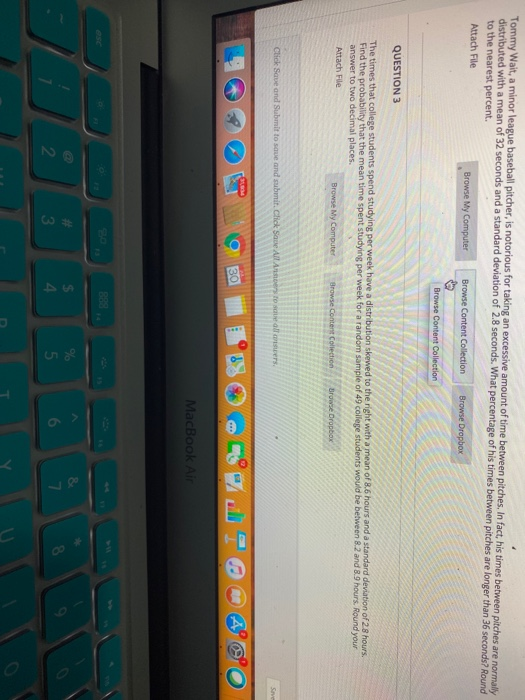Tommy Wait, a minor league baseball pitcher, is notorious for taking an excessive amount of time between pitches. In fact, his times between pitches are normally distributed with a mean of 32 seconds and a standard deviation of 2.8 seconds. What percentage of his times between pitches are longer than 36 seconds? Round to the nearest percent. Attach File Browse My Computer Browse Content Collection Browse Dropbox Browse Content Collection QUESTION 3 The times that college students spend studying per...

• ### UIT WIuld mean of 3.05 and a standard deviation of 0.30. Find the probability that the...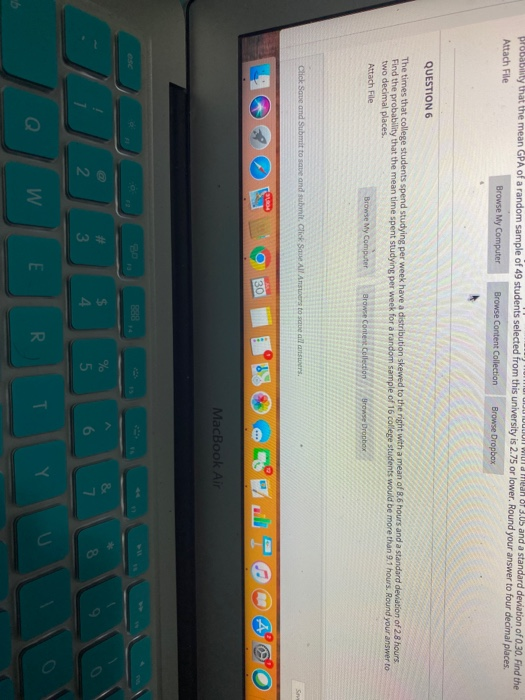UIT WIuld mean of 3.05 and a standard deviation of 0.30. Find the probability that the mean GPA of a random sample of 49 students selected from this university is 2.75 or lower, Round your answer to four decimal places Attach File Browse My Computer Browse Content Collection Browse Dropbox QUESTION 6 The times that college students spend studying per week have a distribution skewed to the right with a mean of 8.6 hours and a standard deviation of 2.8...

• ### A claim is made that college students spend an average of 20 hours per week studying...

A claim is made that college students spend an average of 20 hours per week studying for their courses. You believe the true population average is different than 20 hours, and you would like to test the null hypothesis that μ = 20 hours versus the alternative hypothesis that μ ≠ 20 hours. You gather data from a random sample of 100 college students and calculate a test statistic of 1.2.   This means the p-value would be what? A 0.0576...

• ### A recent survey asked college students how much time they spend studying each week. The results...A recent survey asked college students how much time they spend studying each week. The results showed a mean of 10 hours with a standard deviation of 2 hours. Complete parts (a) through (c) below. (a) Find the probability that a randomly selected student would spend less than 7 hours studying per week. O A. 0.933 OB. 0.500 OC. 0.067 OD. 0.333 (b) What percent of students spend more than 12 hours studying per wook? O A. 80.0% OB. 15.9%...

• ### An educational research group wants to know how many hours college students spend studying outside of...

An educational research group wants to know how many hours college students spend studying outside of class per week. If they survey 100 students and find an average 10.5 hours of studying a week, with a standard deviation of 2.25, find a 98% confidence interval for the true average number of hours spent studying. Answer choices: 10.5±.324 10.5±.225 None of these 10.5±.482 10.5±.524

• ### Assume that the number of hours college students spend working per week is normally distributed with...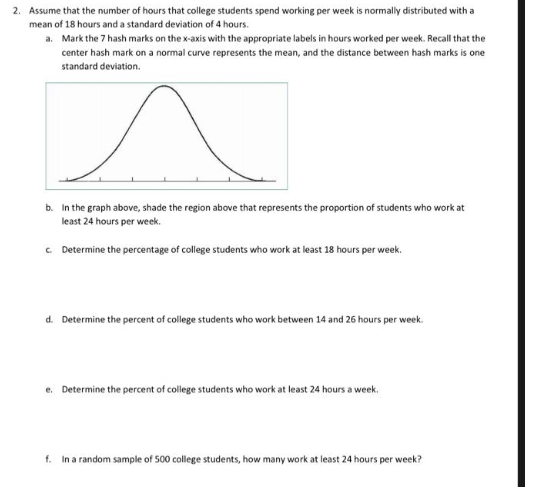Assume that the number of hours college students spend working per week is normally distributed with a mean of 18 hours and standard deviation of 4 hours 2. Assume that the number of hours that college students spend working per week is normally distributed with a mean of 18 hours and a standard deviation of 4 hours. a. Mark the 7 hash marks on the x-axis with the appropriate labels in hours worked per week. Recall that the center hash...